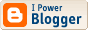## Scooping the Loop Snooper

A proof in verse of the undecidability of the halting problem, in the style of Dr Seuss, by my colleage Geoffrey Pullum.
No general procedure for bug checks succeeds.
Now, I won’t just assert that, I’ll show where it leads:
I will prove that although you might work till you drop,
you cannot tell if computation will stop.

## Proofweb

Looks interesting, but I was unable to figure out how to get it to complete even a simple proof.

## A bizarre function over streams

Alex Simpson showed me this bizarre function. Given a total predicate p over streams, the function (exists p) returns true if there is some stream for which the predicate returns true. This works because if the predicate is total, then there must be some bound on the initial segment of the stream that it examines.

The auxiliary function (epsilon p) returns a stream for which p holds, if one exists, and an arbitrary stream otherwise. The auxiliary function (prefix b p) is like (epsilon p), but only considers streams beginning with b.
`exists :: ([Bool] -> Bool) -> Boolepsilon :: ([Bool] -> Bool) -> [Bool]prefix :: Bool -> ([Bool] -> Bool) -> [Bool]exists p = p (epsilon p)epsilon p = prefix (p (prefix True p)) pprefix b p = b : epsilon (\x -> p (b : x))`
Here are some test predicates and a testing function.
`pos i x = not (x !! i)run i x = and (map not (take i x))alt i x = take i x == take i (cycle [False,True])fal i x = not (take i x == take i x)test f i = (exists (f i), take (i+1) (epsilon (f i)))`
And here are some test runs.
`*Main> test pos 5(True,[True,True,True,True,True,False,True,True])*Main> test run 5(True,[False,False,False,False,False,True,True,True])*Main> test alt 5(True,[False,True,False,True,False,True,True,True])*Main> test fal 5(False,[False,False,False,False,False,False,False,False])`